2012-12-09 15:25:30 love_xunmeng 阅读数 2592
• ###### OpenGL ES2.0 中级篇

16584 人正在学习 去看看 张立铜

I(x,y) = R(x,y)L(x,y); I(x,y)是图像的像素值，R(x,y)是改点的反射率，L(x,y)是改点的光照部分。

L(x,y)变化缓慢，或者说是平滑的。2017-03-16 13:56:00 tinyzhao 阅读数 6025
• ###### OpenGL ES2.0 中级篇

16584 人正在学习 去看看 张立铜

https://en.wikipedia.org/wiki/Spherical_harmonics

## 球谐函数

I(p)=j=19ρ(p)ljhj(p)

ρ(p)$\rho(p)$表示p处的albedo,lj$l_j$表示球谐基函数的系数，hj$h_j$表示9个球谐基函数。使用nx,ny,nz$n_x,n_y,n_z$表示法线方向，这9个基分别为：

h1=14π,h2=34πnz,h3=34πnx

h4=14πny,h5=1254π(3n2z1),h6=3512πnxnz

h7=3512πnynz,h8=32512π(n2xn2y),h9=3512πnxny

## 光照估计

• 初始化albedo估计

albedo的初始值ρ0$\rho^0$可以使用图片像素平均值来估计。

• 初始化光照参数

有了ρ0$\rho^0$，就可以带入到I=ρ0HL$I=\rho^0HL$中，使用最小二乘法获得初始的光照系数L0$L^0$

• 更新albedo

使用上一步中得到的L$L$值，可以根据式子I=ρHL0$I=\rho HL^0$直接解得新的ρ$\rho$

• 更新光照系数

再次使用最小二乘法估计光照系数L$L$。重复估计albedo和L，直到满足要求，一般5次就足够了。

## 总结

2019-08-15 16:29:21 hyk_1996 阅读数 3335
• ###### OpenGL ES2.0 中级篇

16584 人正在学习 去看看 张立铜

## LLNet: A deep autoencoder approach to natural low-light image enhancement

2017 Pattern Recognition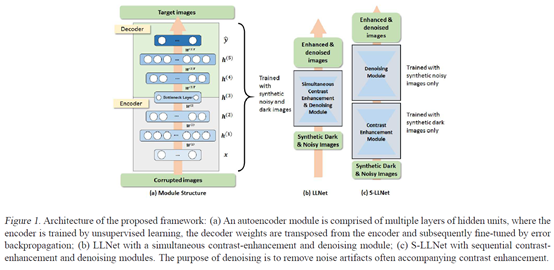（1）我们提出了一种训练数据生成方法（即伽马校正和添加高斯噪声）来模拟低光环境。

（2）探索了两种类型的网络结构：(a) LLNet,同时学习对比度增强和去噪；(b) S-LLNet,使用两个模块分阶段执行对比度增强和去噪。

（3）在真实拍摄到的低光照图像上进行了实验，证明了用合成数据训练的模型的有效性。

（4）可视化了网络权值，提供了关于学习到的特征的insights。

## MSR-net:Low-light Image Enhancement Using Deep Convolutional Network

2017 arXiv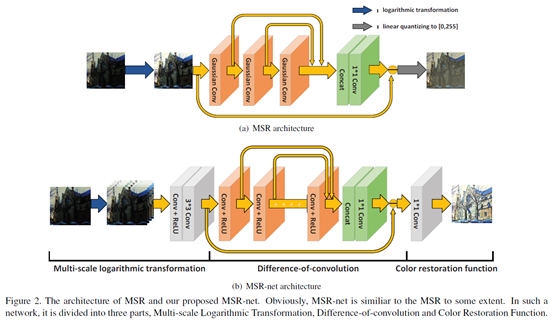## Learning a Deep Single Image Contrast Enhancer from Multi-Exposure Images

2018 TIP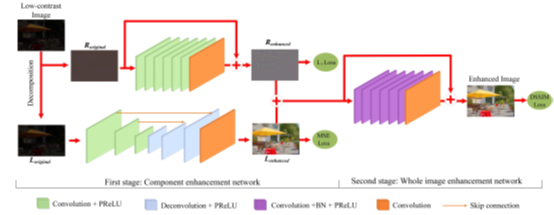（1）构建了一个多曝光图像数据集，包括了不同曝光度的低对比度图像以及对应的高质量参考图像。

（2）提出了一个两阶段的增强模型，如上图所示。第一阶段先用加权最小二乘（WLE）滤波方法将原图像分解为低频成分和高频成分，然后对两种成分分别进行增强；第二阶段对增强后的低频和高频成分融合，然后再次增强，输出结果。

## Deep Retinex Decomposition for Low-Light Enhancement

2018 BMVC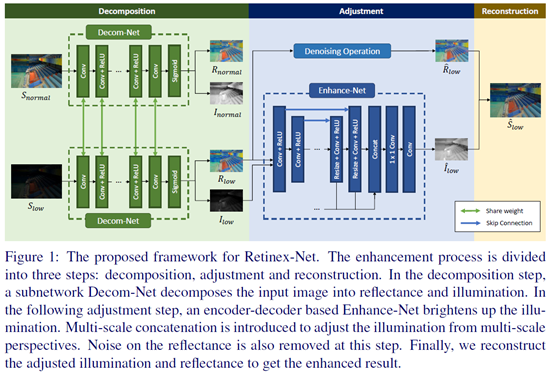（2）提出了Retinex-Net，它分为两个子网络：Decom-Net能够对图像进行解耦，得到光照图和反射图；Enhance-Net对前面得到的光照图进行增强，增强后的光照图和原来的反射图相乘就得到了增强结果。另外，考虑到噪声问题，采用一种联合去噪和增强的策略，去噪方法采用BM3D。

（3）提出一个structure-aware total variation constraint，就是用反射图梯度作为权值对TV loss进行加权，从而在保证平滑约束的同时不破坏纹理细节和边界信息。

## MBLLEN: Low-light Image/Video Enhancement Using CNNs

2018 BMVC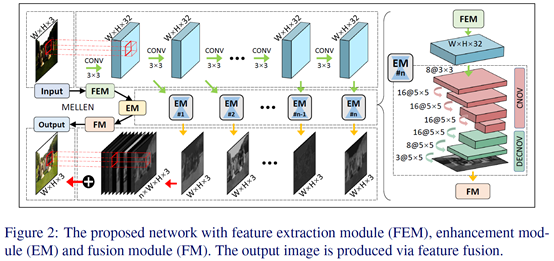2018 CVPR

## Kindling the Darkness: A Practical Low-light Image Enhancer

2019 arXiv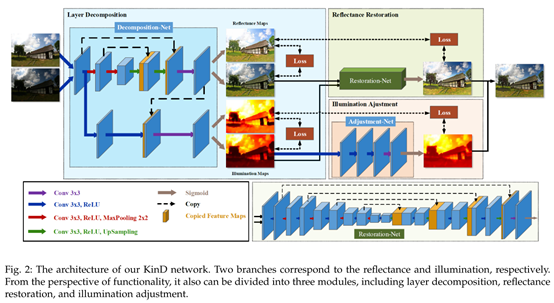（1） 如何有效的从单张图像中估计出光照图成分，并且可以灵活调整光照level?

（2） 在提升图像亮度后，如何移除诸如噪声和颜色失真之类的退化？

（3） 在没有ground-truth的情况下，样本数目有限的情况下，如何训练模型？

（a）对于Decomposition-Net，其损失函数除了沿用Retinex-Net的重构损失和反射图一致损失外，针对光照图的区域平滑性和相互一致性，还增加了两个新的损失函数。

（b）对于Restoration-Net，考虑到了低光照情况下反射图往往存在着退化效应，因此使用了良好光照情况下的反射图作为参考。反射图中的退化效应的分布很复杂，高度依赖于光照分布，因此引入光照图信息。

## 参考文献

 Shi, W., Loy, C. C., & Tang, X. (2016). Deep Specialized Network for Illuminant Estimation. ECCV9908, 371–387. https://doi.org/10.1007/978-3-319-46493-0

 Guo, X., Li, Y., & Ling, H. (2017). LIME: Low-light image enhancement via illumination map estimation. IEEE Transactions on Image Processing26(2), 982–993. https://doi.org/10.1109/TIP.2016.2639450

 Lore, K. G., Akintayo, A., & Sarkar, S. (2017). LLNet: A deep autoencoder approach to natural low-light image enhancement. Pattern Recognition61, 650–662. https://doi.org/10.1016/j.patcog.2016.06.008

 Shen, L., Yue, Z., Feng, F., Chen, Q., Liu, S., & Ma, J. (2017). MSR-net:Low-light Image Enhancement Using Deep Convolutional Network. ArXiv. Retrieved from http://arxiv.org/abs/1711.02488

 Cai, J., Gu, S., & Zhang, L. (2018). Learning a Deep Single Image Contrast Enhancer from Multi-Exposure Images. IEEE Transactions on Image Processing1(c), 1–14. Retrieved from http://www4.comp.polyu.edu.hk/~cslzhang/paper/SICE.pdf

 Gao, Y., Hu, H. M., Li, B., & Guo, Q. (2018). Naturalness preserved nonuniform illumination estimation for image enhancement based on retinex. IEEE Transactions on Multimedia20(2), 335–344. https://doi.org/10.1109/TMM.2017.2740025

 Chen, C., Chen, Q., Xu, J., & Koltun, V. (2018). Learning to See in the Dark. CVPR, 3291–3300. https://doi.org/10.1109/CVPR.2018.00347

 Lv, F., Lu, F., Wu, J., & Lim, C. (2018). MBLLEN: Low-light Image/Video Enhancement Using CNNs. BMVC, 1–13.

 Wei, C., Wang, W., Yang, W., & Liu, J. (2018). Deep Retinex Decomposition for Low-Light Enhancement. BMVC, (61772043). Retrieved from http://arxiv.org/abs/1808.04560

 Zhang, Y., Zhang, J., & Guo, X. (2019). Kindling the Darkness: A Practical Low-light Image Enhancer. ArXiv, 1–13. Retrieved from http://arxiv.org/abs/1905.04161

2019-06-06 13:10:11 weixin_43172215 阅读数 45
• ###### OpenGL ES2.0 中级篇

16584 人正在学习 去看看 张立铜

# 图像增强

Domain)。

## 频率域法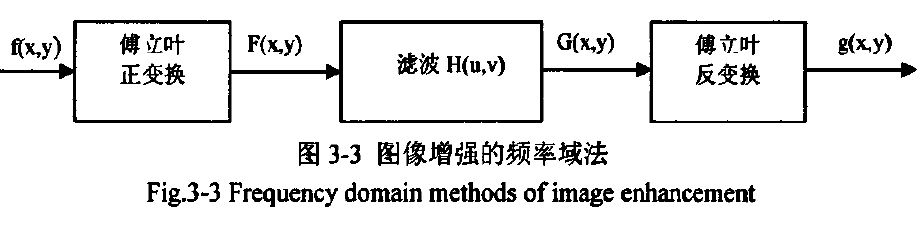图像增强

## 空间域法

### 灰度修正

(1)针对图像某一部分或整幅图像曝光不足而使用的灰度级变换，其目的是增加图

(2)直方图修正，能够使图像具有所需要的灰度分布，从而有选择的突出所需要的

#### a．灰度变换

g(x，y)上每一点像素与f(x，y)上的像素对应相同的坐标位置。算子@有 线性，分段线性

l、直方图概念

（具体公式要再看）

a，均值滤波 b．中值滤波

# 图像分割（特征提取？？）

## a．边缘检测的梯度算法

l、梯度边缘检测算法基本步骤

(1)滤波：边缘检测算法主要是基于图像强度的一阶和二阶导数，但导数的计算对噪声敏感，因此必须使用滤波器来改善与噪声有关的边缘检测器的性能。需要指出，大多数滤波器在降低噪声的同时也导致了边缘强度的损失，因此，增强边缘和降低噪声之间需要折中。
(2)增强：增强边缘的基础是确定图像各点领域强度的变化值。增强算法可以将领域(或局部)强度值有显著变化的点突显出来。边缘增强一般是通过计算梯度来完成的。
(3)检测：在图像中有许多点的梯度幅值比较大，而这些点在特定的应用领域中并不都是边缘，所以应该用某种方法来确定哪些点是边缘点。最简单的边缘检测判据是梯度幅值阈值判断。
(4)定位：如果某一应用场合要求确定边缘位置，则边缘检测的位置可在子像素分辨率上来估计，边缘的方位也可以被估计出来。

## b 灰度阈值分割

(1)基于各像素值得阈值阈值仅根据f(x，Y)来选取，所得到的阈值仅与全图各

(2)基于区域性质的阈值阈值是根据f(x，Y)和p(x，Y)来选取的，所得的阈值与

(3)基于坐标位置的阈值阈值进一步(除根据f(x，y)和p(x，y)来选取外)还与x，Y

l、极小值点阈值

2、最优阈值

### 动态阈值

l、阈值插值

(1)将整幅图像分成一系列互相之间有50％重叠的子图像；
(2)做出每个子图像的直方图；
(3)检测各个子图像的直方图是否为双峰，如果是就进行阈值选取，否则不处理；
(4)根据对直方图为双峰的子图像得到的阈值，通过插值得到所有的子图像的阈值：
(5)根据各子图像的阂值，再通过插值得到所有像素的阂值，然后对图像进行分割。
2、水线阈值算法

# 总结

(1)由于诸多原因造成实验所得到的工件图像只有中间区域可用，所以介绍了工件图像的剪切方法，并简要介绍了工件图像光照不均匀校正的有效方法。
(2)在图像增强方法中，主要包括灰度修正(改善对比度)和图像平滑(消噪)两方面，通过对工件图像进行具体算法处理，比较之后得到了适用于工件图像增强的最佳办法。在实际应用中，我们选用灰度变换的方法来改善工件图像的对比度，选用中值滤波的方法对工件图像进行消噪。
(3)在图像分割方法中，简要地介绍了图像分割方法的基本概念，并对工件图像进行了边缘检测和灰度闽值分割算法的处理，结果比较之后发现在进行边缘检测时，Canny算子的检测结果最好，它能把比较细节的边缘检测出来，另外在对工件图像进行阈值分割时，最佳的方法为全局阈值法。

# 纹理分析的基本方法介绍

(1)角二阶矩E(能量)
(2)惯性矩I(对比度)
(3)熵H
(4)局部均匀性工(逆差矩)

## 等灰度游程长度法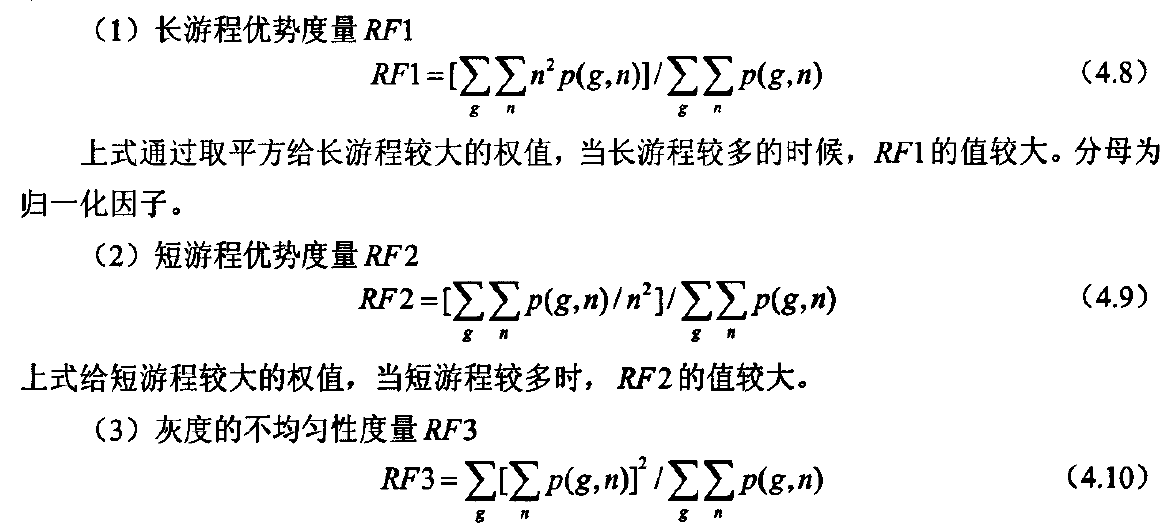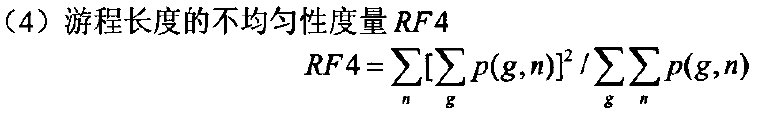1. 全新的界面设计 ，将会带来全新的写作体验；
2. 在创作中心设置你喜爱的代码高亮样式，Markdown 将代码片显示选择的高亮样式 进行展示；
3. 增加了 图片拖拽 功能，你可以将本地的图片直接拖拽到编辑区域直接展示；
4. 全新的 KaTeX数学公式 语法；
5. 增加了支持甘特图的mermaid语法1 功能；
6. 增加了 多屏幕编辑 Markdown文章功能；
7. 增加了 焦点写作模式、预览模式、简洁写作模式、左右区域同步滚轮设置 等功能，功能按钮位于编辑区域与预览区域中间；
8. 增加了 检查列表 功能。

H2O is是液体。

210 运算结果是 1024.

## 如何插入一段漂亮的代码片

// An highlighted block
var foo = 'bar';


• 项目
• 项目
• 项目
1. 项目1
2. 项目2
3. 项目3
• 计划任务
• 完成任务

## 创建一个表格

### SmartyPants

SmartyPants将ASCII标点字符转换为“智能”印刷标点HTML实体。例如：

TYPE ASCII HTML
Single backticks 'Isn't this fun?' ‘Isn’t this fun?’
Quotes "Isn't this fun?" “Isn’t this fun?”
Dashes -- is en-dash, --- is em-dash – is en-dash, — is em-dash

## 创建一个自定义列表

Markdown
Text-to-HTML conversion tool
Authors
John
Luke

## 注释也是必不可少的

Markdown将文本转换为 HTML

## KaTeX数学公式

Gamma公式展示 $\Gamma(n) = (n-1)!\quad\forall n\in\mathbb N$ 是通过欧拉积分

$\Gamma(z) = \int_0^\infty t^{z-1}e^{-t}dt\,.$

## 新的甘特图功能，丰富你的文章

• 关于 甘特图 语法，参考 这儿,

## UML 图表

• 关于 Mermaid 语法，参考 这儿,

## FLowchart流程图

• 关于 Flowchart流程图 语法，参考 这儿.

## 导出与导入

### 导入

1. 注脚的解释 ↩︎

2014-07-02 19:09:10 u013308233 阅读数 769
• ###### OpenGL ES2.0 中级篇

16584 人正在学习 去看看 张立铜

Retinex理论是Land提出的从信号分析角度出发，直接从单张图像中提取光照不变量。

Retinex方法主要包括两部分：关照的估计和归一化。

//INFaceTool.h
#include<opencv2\opencv.hpp>
using namespace cv;
Mat normalize8(Mat &X,int model=1);
// The function applies the adaptive single scale retinex algorithm to an image.
//This is an auxialry function for computing the iterative convolution
Mat convolute(Mat &X,Mat&y,Mat&N);


//INFaceTool.cpp
#include<opencv2\opencv.hpp>
#include<core\core.hpp>
#include"INFaceTool.h"
using namespace cv;
//The function adjusts the dynamic range of the grey scale image to the interval [0,255] or [0,1]
Mat normalize8(Mat&X,int model)
{
double max_v_x;
double min_v_x;
Mat Y(X.rows,X.cols,CV_64F);
Mat Ones=Mat::ones(X.rows,X.cols,CV_64F);
minMaxLoc(X,&min_v_x,&max_v_x,NULL,NULL);
if(model==1)
{
Y=((X-min_v_x*Ones)/(max_v_x*Ones-min_v_x*Ones))*255;
int nl=Y.rows;
int nc=Y.cols;
if(Y.isContinuous())
{
nc=nc*nl;
nl=1;
}
for(int j=0;j<nl;j++)
{
double*data=Y.ptr<double>(j);
for(int i=0;i<nc;i++)
{
//std::cout<<"data"<<data[i];
data[i]=ceil(data[i]);

}
}
return Y;
}
else
{
Y=(X-min_v_x*Ones)/(max_v_x*Ones-min_v_x*Ones);
return Y;
}

}
{
Mat X=img.clone();
if(X.channels()==3)
{
cvtColor(X,X,CV_RGB2GRAY);
}

X.convertTo(X,CV_64F);
X=normalize8(X);
// Compute spatial gradient in x and y directions
int a=X.rows;
int b=X.cols;
Mat X1=Mat::zeros(a,b+2,CV_64F);
Mat X1r=X1(Rect(2,0,b,a)); X.copyTo(X1r);
Mat X2=Mat::zeros(a,b+2,CV_64F);
Mat X2r=X2(Rect(0,0,b,a));X.copyTo(X2r);
Mat Gx=X1(Rect(1,0,b,a))-X2(Rect(1,0,b,a));
X1=Mat::zeros(a+2,b,CV_64F);
X1r=X1(Rect(0,2,b,a));X.copyTo(X1r);
X2=Mat::zeros(a+2,b,CV_64F);
X2r=X2(Rect(0,0,b,a));X.copyTo(X2r);
Mat Gy=X1(Rect(0,1,b,a))-X2(Rect(0,1,b,a));
pow(Gx,2,Gx);
pow(Gy,2,Gy);
Mat I;
pow((Gx+Gy),0.5,I);

//Compute local inhomogenity
Mat tao=Mat::zeros(a,b,CV_64F);
Mat Xtmp=Mat::zeros(a+2,b+2,CV_64F);
Mat Xtmpr=Xtmp(Rect(1,1,b,a));X.copyTo(Xtmpr);
float suma=0;
for(int i=1;i<a+1;i++)
{
for(int j=1;j<b+1;j++)
{
suma=0;
for(int k=-1;k<2;k++)
{ for(int h=-1;h<2;h++)
{
suma=suma+abs(X.at<double>(i-1,j-1)-Xtmp.at<double>(i+k,j+h));
}
}
suma=suma/9;
tao.at<double>(i-1,j-1)=suma;

}
}
double tao_min,tao_max;
minMaxLoc(tao,&tao_min,&tao_max,NULL,NULL);
Mat tao_slash=(tao-tao_min)/(tao_max-tao_min);
int nl=tao_slash.rows;
int nc=tao_slash.cols;
if(tao_slash.isContinuous())
{
nc=nc*nl;
nl=1;
}
for(int j=0;j<nl;j++)
{
double*data=tao_slash.ptr<double>(j);
for(int i=0;i<nc;i++)
{
//std::cout<<"data"<<data[i];
data[i]=sin(CV_PI/2*data[i]);

}
}

Scalar m1=mean(I);
Scalar m2=mean(tao_slash);
double meanI=m1.val;
double meanH=m2.val;
//Set needed parameters if they are not provided as inputs
double S=10*exp(-(meanI)/10);
double h=0.1*exp(-(meanH)/0.1);

//Determine weight functions
Mat sqrt_tao_slash;
Mat sqrt_I;
pow(tao_slash/h,0.5,sqrt_tao_slash);
pow(I/S,0.5,sqrt_I);
Mat alpha=1/(1+sqrt_tao_slash);
Mat beta=1/(1+sqrt_I);
Mat weight=alpha.mul(beta);
//precompute Ns
Mat w=Mat::zeros(a+2,b+2,CV_64F);
Mat wr=w(Rect(1,1,b,a));
weight.copyTo(wr);
Mat N=Mat::zeros(a,b,CV_64F);
for(int i=1;i<a+1;i++)
{

for(int j=1;j<b+1;j++)
{   suma=0;
for(int k=-1;k<2;k++)
{
for(h=-1;h<2;h++)
{
suma=suma+(w.at<double>(i+k,j+h));
}
}
N.at<double>(i-1,j-1)=suma;
}
}
// Start iterative convolution
Mat L_old;
X.copyTo(L_old);
Mat L_new,L_new_s;
L_new=Mat::zeros(a,b,CV_64F);
L_new_s=Mat::zeros(a,b,CV_64F);
for(int i=0;i<T;i++)
{
L_new_s=convolute(L_old,weight,N);
L_new=max(L_new_s,L_old);
L_new.reshape(0,a);
L_new.copyTo(L_old);
}

//Produce ilumination invariant representation of input image
nc=X.cols;
nl=X.rows;
if(X.isContinuous())
{
nc=nc*nl;
nl=1;
}
// R=Mat::zeros(a,b,CV_64F);
L=Mat::zeros(a,b,CV_64F);;
for(int j=0;j<nl;j++)
{ //double*dataR=R.ptr<double>(j);
double*dataX=X.ptr<double>(j);
double*dataL=L.ptr<double>(j);
double*dataL_new=L_new.ptr<double>(j);
for(int i=0;i<nc;i++)
{
//dataR[i]=log(dataX[i]+1)-log(dataL_new[i]+1);
dataL[i]=log(dataL_new[i]+1);

}
}

L=normalize8(L);
// R=normalize8(R);
L.convertTo(L,CV_8U);
//R.convertTo(R,CV_8U);
I.convertTo(I,CV_8U);
tao_slash.copyTo(tao_slash);
//tao_slash.copyTo(LocalInhomogenity);

}
//This is an auxialry function for computing the iterative convolution
Mat convolute(Mat &X,Mat&y,Mat&N)
{
int a=X.rows;
int b=X.cols;
Mat X1=Mat::zeros(a+2,b+2,CV_64F);
Mat X1r=X1(Rect(1,1,b,a));X.copyTo(X1r);

Mat w=Mat::zeros(a+2,b+2,CV_64F);
Mat wr=w(Rect(1,1,b,a));y.copyTo(wr);
Mat Y=Mat::zeros(a,b,CV_64F);
double tt;
for(int i=1;i<a;i++)
{
for(int j=1;j<b;j++)
{

tt=sum(X1(Rect(j-1,i-1,3,3)).mul(w(Rect(j-1,i-1,3,3)))).val;
Y.at<double>(i-1,j-1)=tt/N.at<double>(i-1,j-1);

}
}
return Y;

}